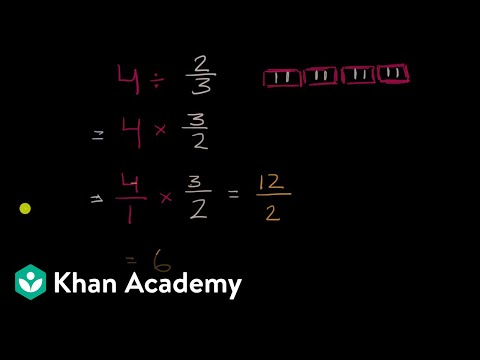Video

# Dividing a fraction by a whole number (Full video)

Description: Sal uses an area model to divide a fraction by a whole number. Let's see if we can figure out what 2/3 divided by five is equal to. Now, I can divide into three equal sections. Well, the way I could do this is I could divide it into five equal sections.

### Other videos you might be interested in### Dividing Fractions Word Problems (Full video)

#### Student Achievement Partners